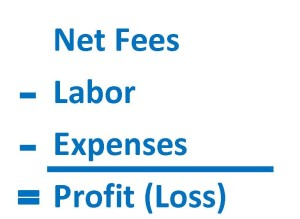# Profit Margin

What Does It Mean?

The most basic and important key indicator, Profit Margin can be stated in either dollar or percentage terms.  Profit is calculated for a specific accounting period, whether for the month, the year to date (YTD), or for the entire fiscal year.

The Simple Math

To arrive at profit in dollar terms, simply subtract ALL of your expenses for the accounting period from your Net Fees (Net Revenues) for that same period. Expenses are typically broken down between Labor and Expense, giving us this simple calculation:To arrive at Profit Margin in percentage terms, merely divide the total profit for the period by the total Net Fees for the period:

Profit (%) = Profit (\$) / Net Fees (\$)

The Big Idea

Profit is the primary financial goal of your business (unless you are a non-profit, of course, and then you might refer to this as “surplus”).  Your for-profit business really must make a profit, and Profit Margin is the very first thing we should consider upon completion of any accounting period.  Consistent, healthy profit levels mean a stable business.

Taking Control

As the math would suggest, profit has three primary levers: Net Fees, Labor, and Expense.  Profit is maximized by increasing Net Fees, and conversely, reducing, or at least controlling, Labor and Expense costs.